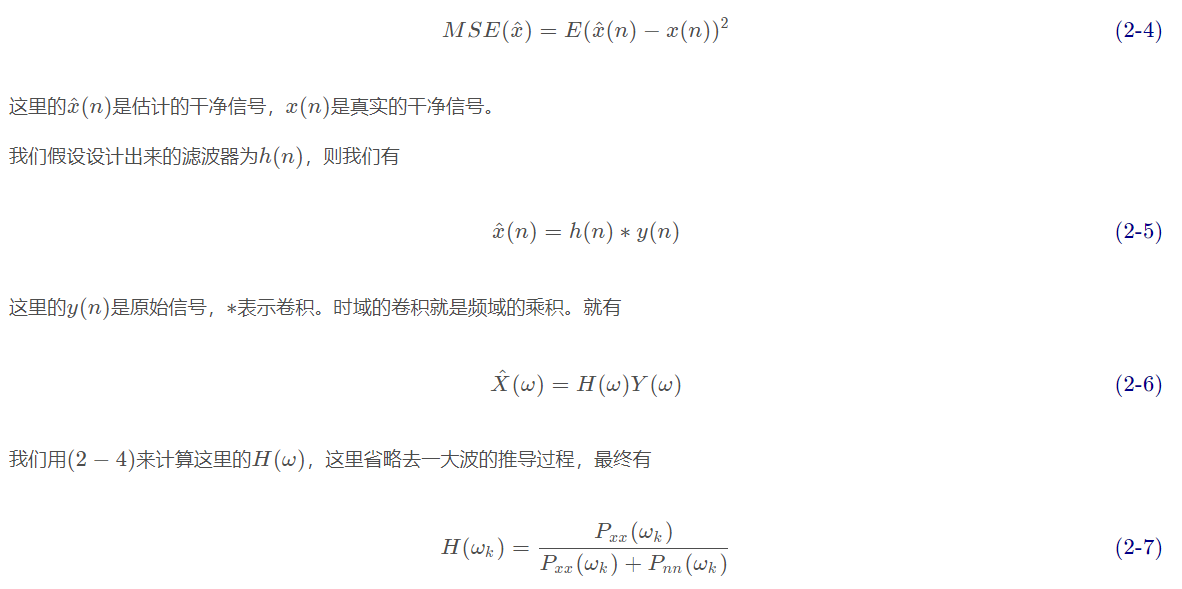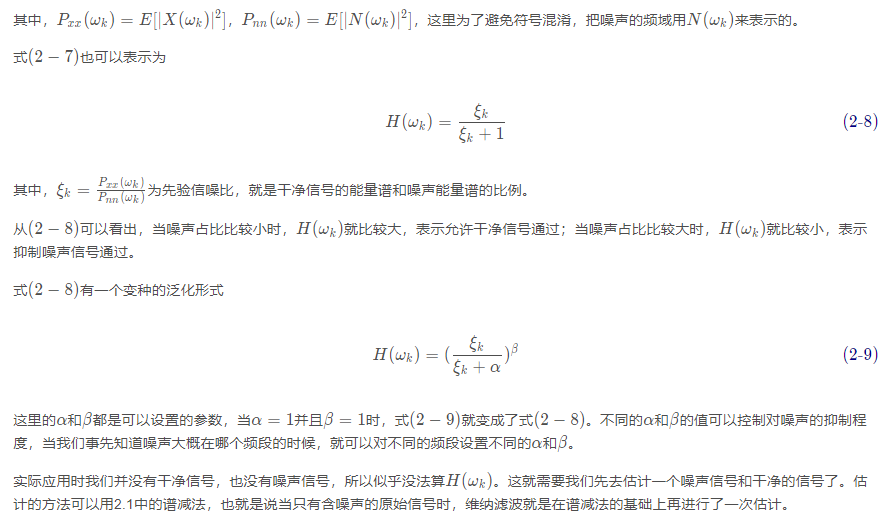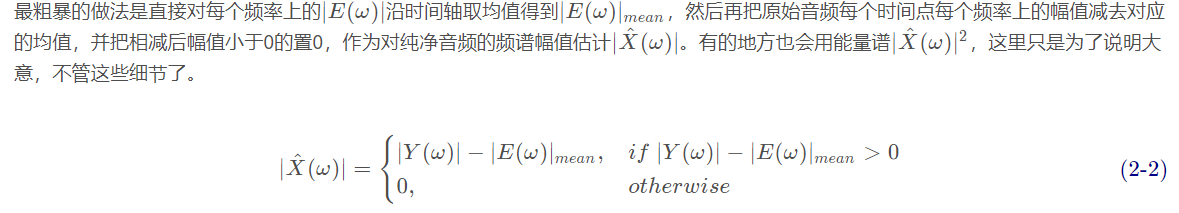# 【语音去噪】基于matlab谱减法+最小均方+维纳滤波语音去噪【含Matlab源码 1542期】

## 一、简介

1 维纳滤波法2 卡尔曼

3 谱减法

noisereduce中的stationary的方法就是用谱减法去做的，效果还是不错的，不过也只能应对于stationary noise。

（1）截取头部一小段语音作为噪声
e(m)=y(n)[:m]

（2）分别计算原始音频和噪声的STFT，Y(ω)和E(ω)。
（3）根据噪声的频谱幅值，对原始音频的频谱幅值进行谱减。noisereduce中的具体实现略有不同，它过减用∣E(ω)∣的方差来控制，一般是1.5倍或者1.0倍的方差。代码片段如下所示

self.mean_freq_noise = np.mean(noise_stft_db, axis=1)
self.std_freq_noise = np.std(noise_stft_db, axis=1)
self.noise_thresh = self.mean_freq_noise + self.std_freq_noise * self.n_std_thresh_stationary


（4）对∣ X ^ ( ω ) ∣做平滑处理，使得声音失真没那么严重。
noisereduce中使用的scipy.signal.fftconvolve来实现这一过程。
（5）结合原始音频的相位，还原谱减后的音频。这就是个反向STFT的过程。

## 二、部分源代码

global hr1 hr2 hr3 hr4 s y fs
clf reset
set(gcf,'unit','nor malized','position',[0.1,0.1,0.85,0.85]);
set(gcf,'defaultuicontrolunits','normal')

hr2=uicontrol(gcf,'style','toggle','string','开始/关闭','position',[0.72,0.65,0.15,0.05]);
%uicontrol(gcf,'style',')
%htitle1=title('原是语音波形');
uicontrol('style','text','string','原始语音波形','position',[0.25,0.93,0.12,0.03],'horizontal','center');
h_axes1=axes('position',[0.05,0.54,0.52,0.38]);
set(h_axes1,'ylim',[-1,1]);
%t=0:pi/50:2*pi;
%y=sin(t);
%plot(t,y);
[y,fs,bit]=wavread('D:\3 ★Matlab★\Matalb CSDN\【信号处理】\【语音处理】\【语音增强】基于matlab谱减法、最小均方和维纳滤波语音增强【含Matlab源码 482期】\1_NumSound_snr10.wav');
L1=length(y);
t1=1:L1;
plot(t1,y);

uicontrol('style','text','string','增强后语音波形','position',[0.25,0.45,0.12,0.03],'horizontal','center');
h_axes2=axes('position',[0.05,0.05,0.52,0.38]);
set(h_axes2,'ylim',[-1,1]);
set(hr1,'callback','speech_enhancement');
set(hr2,'callback','speech_enhancement');

hr3=uicontrol(gcf,'style','toggle','string','播放原始语音','position',[0.7,0.5,0.18,0.05],'horizontal','center');
hr4=uicontrol(gcf,'style','toggle','string','播放增强后的语音','position',[0.7,0.42,0.18,0.05],'horizontal','center');
set(hr3,'callback','play');
set(hr4,'callback','play');
function  y=check(x,n);
E=0;
for i=1:n,
E=x(i)*x(i)+E;
end;
E=E/n;
if E<0.01,
x=zeros(1,n);
else
x=x;
end;
y=x;
figure(1);
plot(wave_serial);

b=fir1(10,1/8);
s=filter(b,1,wave_serial);

E=zeros(1,2000);
N=2000;
for i=1:N
E(i)=abs(s(i));
end
figure(2);
plot(E);

M1=0.03;
for i=0:N
if E(N-i)>M1
X=N-i;
break
end
end

X1=X-600;
X2=X-200;
s1=s(X1:X2);
N1=200;
r=zeros(1,200);
for i=1:N1
for j=1:N1
r(i)=r(i)+abs(s1(j)-s1(i+j));
end
end
figure(3);
plot(r);

M2=r(1);
for i=1:N1
if r(i)>M2
M2=r(i);
end
end
M2=M2/2-1;

Y=zeros(1,20);
B=zeros(1,20);
j=0;
for i=2:(N1-1)
if r(i-1)>r(i) & r(i)<r(i+1) & r(i) <M2
j=j+1;
Y(j)=i;
B(j)=r(i);
end
end

F=0;
j=j-1;
N_keynote=0;
for i=1:j
N_keynote=N_keynote+(Y(i+1)-Y(i));
end
F=j*4000/N_keynote;

res='0';
if F<=195
res='M';
else
res='F';
end

F
M2
res
Y
B
[Y,f]=psd(y(1500:1660),256,8000);
plot(f,Y,'r-');


## 三、运行结果## 四、matlab版本及参考文献

1 matlab版本
2014a

2 参考文献
韩纪庆,张磊,郑铁然.语音信号处理（第3版）[M].清华大学出版社，2019.
柳若边.深度学习:语音识别技术实践[M].清华大学出版社，2019.

07-30298
07-063027
09-032601
11-201564
06-14782
05-31154
03-19938
03-192637
05-187万+
03-16467
06-16310
05-2241
01-193369
01-031041
04-05510

### “相关推荐”对你有帮助么？

•非常没帮助
•没帮助
•一般
•有帮助
•非常有帮助

©️2022 CSDN 皮肤主题：Age of Ai 设计师：meimeiellie被折叠的  条评论 为什么被折叠?到【灌水乐园】发言¥2 ¥4 ¥6 ¥10 ¥20余额支付 (余额：-- )扫码支付获取中扫码支付点击重新获取扫码支付1.余额是钱包充值的虚拟货币，按照1:1的比例进行支付金额的抵扣。
2.余额无法直接购买下载，可以购买VIP、C币套餐、付费专栏及课程。余额充值